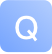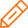# C#编程实现一个冒泡排序算法-问答-阿里云开发者社区-阿里云## C#编程实现一个冒泡排序算法

2018-07-16 11:23:56 1613 5取消 提交回答

• 沉默术士
2019-07-17 22:50:55
int[] scores=new int; //假设有5个元素的数组
int i,j; //循环变量
int temp; //临时变量

for(i=0;i<scrores.Length-1;i++)//控制比较多少轮
{
for(j=0;j<scrores.Length-1-i;j++)//将最大的元素交换到最后
{
if(scores[j]>scroes[j+1])
{ //交换元素
temp=scroes[j];
scroes[j]=csroes[j+1];
scroes[j+1]=temp;
}
}
}

-------------------------

int[] a=new int{5,9,7,3,8};
int tmp=0;
for(int i=0;i<a.length-1;i++)
{
for(int j=i+1;j<a.length;j++)
{
if(a[i]<a[j])
{
tmp=a[i];
a[i]=a[j];
a[j]=tmp;
}
}
}

0 0
• 知与谁同
2019-07-17 22:50:55
public int[] Sort1(int[] item)
{
int i, j;
int temp;
for (i = 0; i < item.length; i++) {
for (j = 0; j < item.length - i - 1; j++) {
if (item[j] > item[j + 1]) {
temp = item[j];
item[j] = item[j + 1];
item[j + 1] = temp;
}
}
}
return item;
}

在网吧写的 没有调试 你试试
0 0
• 管理贝贝
2019-07-17 22:50:55
int[] l = { 5,3,7,1,0,9};//测试的数组
for (int i = 0; i <l.Length - 1; i++)
{
for (int j = 0; j < l.Length - i -1; j++)
{
//升续
if (l[j] > l[j + 1])
{
int temp = l[j];
l[j] = l[j + 1];
l[j + 1] = temp;
}

//降续
/*
if (l[j] < l[j + 1])
{
int temp = l[j];
l[j] = l[j + 1];
l[j + 1] = temp;
}
*/
}
}
0 0
• 一键天涯
2019-07-17 22:50:55
public int[] maoPao(int[] list)//冒泡排序
{
int i, j, temp;

bool done = false;

j = 1;

while ((j < list.Length) && (!done))
{
done = true;

for (i = 0; i < list.Length - j; i++)
{

if (list[i] > list[i + 1])
{

done = false;

temp = list[i];

list[i] = list[i + 1];

list[i + 1] = temp;

}
}
j++;
}
return list;
}
0 0
• 琴瑟
2019-07-17 22:50:55
using System;
using System.Collections.Generic;
using System.Text;

namespace test11
{
class Program
{
const int N = 10;

static void Main(string[] args)
{
int[] a=new int[N];

for (int i = 0; i < N; i++)

for(int i=0;i<N-1;i++)
for (int j = N - 2; j >= i; j--)
{
if (a[j+1] < a[j])
{
int t;
t = a[j+1];
a[j+1] = a[j];
a[j] = t;

}

}
for (int i = 0; i < N; i++)
Console.WriteLine("{0}", a[i]);

}
}
}
0 0添加回答

79

qilu 2014-06-18 15:37:00 73574浏览量 回答数 79

10

【精品问答合集】Hbase热门问答

hbase小能手 2019-05-29 14:37:26 123240浏览量 回答数 10

19

【分享】如何提高网站的打开速度？

enj0y 2012-11-17 14:35:22 57819浏览量 回答数 19

38

19

【分享】如何提高网站的打开速度？

enj0y 2012-11-17 14:35:22 47709浏览量 回答数 19

15

yundun1 2012-02-24 17:40:01 47794浏览量 回答数 15

3

2020年05月编程排行榜-C语言继2015年，重新成为编程排行榜第一名

huc_逆天 2020-05-06 13:43:42 36833浏览量 回答数 3

58

13

11

Windows2008r2+IIS7.5+mysql+php+URL_rewrite伪静态的环境配置

zhedianshi 2014-07-23 10:23:25 48015浏览量 回答数 11
10076

2994

JS零基础入门教程（上册）